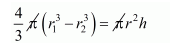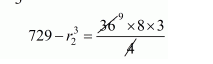# A spherical shell of lead,`
Question:

A spherical shell of lead, whose external diameter is 18 cm, is melted and recast into a right circular cylinder, whose height is 8 cm and diameter 12 cm. Determine the internal diameter of the shell.

Solution:

$r_{1}=\frac{18}{2}=9 \mathrm{~cm}$

Let r2 be the internal radius of spherical shell.

Height of right circular cylinder h = 8 cm

& radius of right circular cylinder

$r=\frac{12}{2}=6 \mathrm{~cm}$

Clearly, volume of spherical shell = volume of right circular cylinder$\frac{4}{3}\left(9^{3}-r_{2}^{3}\right)=(6)^{2} \times 8$$729-r_{2}^{3}=216$

$r_{2}^{3}=513$

$r_{2}=\sqrt{513}$

$r_{2}=3(19)^{1 / 3} \mathrm{~cm}$